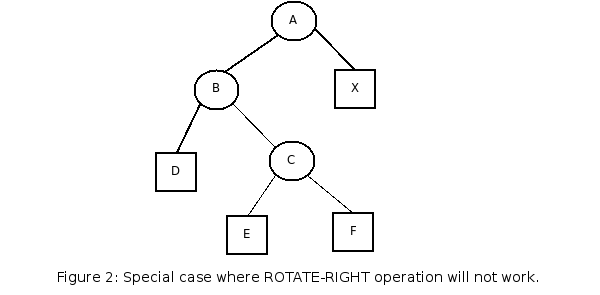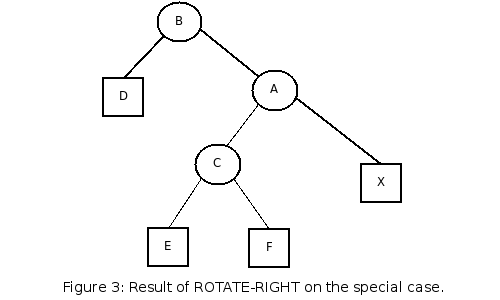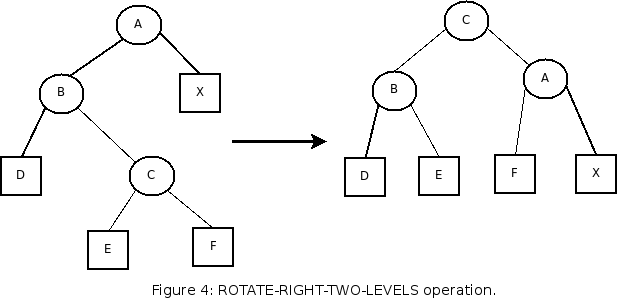# AVL Trees

AVL trees are a kind of balanced binary trees. Insertion and deletion from the tree is done like in a normal binary tree, but the operations are followed by a special rebalance operation.

The balance of the tree is kept by storing the height of each node and comparing left and right childrens' heights to determine whether a rotation is necessary or not.

A rotation is necessary when the height difference between the two childs of a node is greater than 1. For instance, if the left child of a node is 6 links high and the right child is 4 links high, then we can make a new subtree by rotating the subtree to right, resulting in two 5-high children. This operation is illustrated below:In this illustration, letters in circles denote single nodes and letters in boxes denote subtrees, which can possibly be null.

This operation by itself is not enough to maintain a balance in all cases, though. Consider the following situation:This case can be achieved by, for instance, inserting E when F was null. Making a right rotation in this case will not re-balance the tree at all:The operation has simply unbalanced the tree in the other direction. When we encounter such situations, we instead do the following:Choosing between a ROTATE-RIGHT and ROTATE-RIGHT-TWO-LEVELS is done by looking at the children of B. If B is left-heavy, then a ROTATE-RIGHT will not cause a new unbalance because the right child of B is not higher than the left child (see FIGURE 1). If B is right-heavy, then a ROTATE-RIGHT-TWO-LEVELS is necessary. The REBALANCE operation simply calculates the height of a node after an insertion or deletion, and then does the rotations, if any.
```  REBALANCE (A):
calculate_height (A);
P= parent of A.
U= height( A.left ) - height( A.right )
if (U<-1)
if left_heavy(A.right)
rotate_left_twolevels(A)
else
rotate_left(A)
else if (U>1)
if right_heavy(A.left)
rotate_right_twolevels(A)
else
rotate_right(A)
rebalance(P);
```
As you can see, REBALANCE works up to the top of the tree.
```    -[ c library "avl.c", header "avl.h" ]
-[public] typedef struct anode {
struct anode *left, *right, *parent;
int height;
int data;
} anode_t;
-[public] typedef struct {
anode_t *root;
} atree_t;
-[public] void atree_insert (atree_t * T, int data)
{
node_t *N;
anode_t *P;
N = calloc (1, sizeof (*N));
N->data = data;
N->height = 1;
if (!T->root)
{
T->root = N;
return;
}
P = T->root;
while (1)
{
if (N->data < P->data)
{
if (P->left)
P = P->left;
else
{
P->left = N;
N->parent = P;
break;
}
}
else if (N->data > P->data)
{
if (P->right)
P = P->right;
else
{
P->right = N;
N->parent = P;
break;
}
}
else
{
free (N);
return;
}
}
rebalance (T, N);
}
-[private] void calculate_height (anode_t * N)
{
int L, R;
L = theight (N->left);
R = theight (N->right);
if (L > R)
N->height = L + 1;
else
N->height = R + 1;
}
-[private] void rebalance (atree_t * T, anode_t * N)
{
int u;
anode_t *P;
calculate_height (N);
u = theight (N->left) - theight (N->right);
P = N->parent;
if (u < -1) {
if (left_heavy (N->right))
rotate_left_twolevels (T, N);
else
rotate_left (T, N);
} else if (u > 1) {
if (right_heavy (N->left))
rotate_right_twolevels (T, N);
else
rotate_right (T, N);
}
if (P)
rebalance (T, P);
}
-[private] void replace_child_in_parent
(atree_t * T, anode_t * old, anode_t * nw)
{
if (old->parent) {
if (old->parent->left == old)
old->parent->left = nw;
else
old->parent->right = nw;
} else {
T->root = nw;
}
}
-[private] void rotate_right
(atree_t * T, anode_t * A)
{
anode_t *B;
B = A->left;
A->left = B->right;
if (A->left)
A->left->parent = A;
B->parent = A->parent;
replace_child_in_parent (T, A, B);
B->right = A;
A->parent = B;
calculate_height (A);
calculate_height (B);
}
-[private] void rotate_right_twolevels
(atree_t * T, anode_t * A)
{
anode_t *B, *C;
B = A->left;
C = B->right;
/* we know that B and C are non-null since
A was left heavy and B was right heavy */
A->left = C->right;
if (A->left)
A->left->parent = A;
B->right = C->left;
if (B->right)
B->right->parent = B;
C->parent = A->parent;
replace_child_in_parent (T, A, C);
C->left = B;
C->right = A;
A->parent = C;
B->parent = C;
calculate_height (A);
calculate_height (B);
calculate_height (C);
}
-[private] int right_heavy (anode_t * A)
{
if (!A) return 0;
return (theight (A->right) - theight (A->left)) > 0;
}
-[private] void
rotate_left (atree_t * T, anode_t * A)
{
anode_t *B;
B = A->right;
A->right = B->left;
if (A->right)
A->right->parent = A;
B->parent = A->parent;
replace_child_in_parent (T, A, B);
B->left = A;
A->parent = B;
calculate_height (A);
calculate_height (B);
}
-[private] void rotate_left_twolevels
(atree_t * T, anode_t * A)
{
anode_t *B, *C;
B = A->right;
C = B->left;
/* we know that B and C are non-null since
A was right heavy and B was left heavy */
A->right = C->left;
if (A->right)
A->right->parent = A;
B->left = C->right;
if (B->left)
B->left->parent = B;
C->parent = A->parent;
replace_child_in_parent (T, A, C);
C->right = B;
C->left = A;
A->parent = C;
B->parent = C;
calculate_height (A);
calculate_height (B);
calculate_height (C);
}
-[private] int
left_heavy (anode_t * A)
{
if (!A) return 0;
return (theight (A->left) - theight (A->right)) > 0;
}
-[private] int theight (anode_t * A)
{
if (A) return A->height;
else return 0;
}